# College Physics : Ideal Gases

## Example Questions

### Example Question #1 : Ideal Gases

If an ideal gas has its temperature doubled while its volume is cut in half, what happens to its pressure?

The pressure increases by a factor ofThe pressure increases by a factor ofThere is no change in the pressure

The pressure decreases by a factor ofThe pressure increases by a factor ofExplanation:

In this question, we're told that an ideal gas undergoes a change in its temperature and volume, and we're asked to determine how its pressure changes.

First, we'll need to recall the ideal gas equation.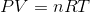Then, we can rearrange to isolate the term for pressure.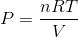From this expression, we can see that if we double the volume, the pressure will double. Also, cutting the volume in half would double the pressure as well. Therefore, doing both of these would cause the pressure to increase by a factor of.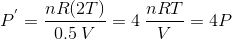### Example Question #1 : College Physics

What volume does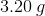of oxygen gas occupy at STP?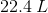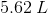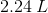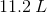Explanation:

The first step in this question is to convert the mass of oxygen given into moles.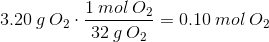Next, it's important to recognize that we'll need to use the ideal gas equation. Since we're told that the gas is under STP (standard temperature and pressure), we have everything we need to solve for volume.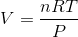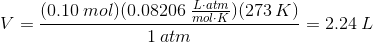### All College Physics Resources